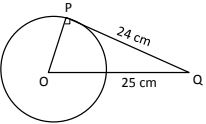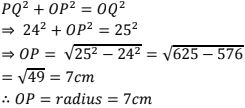# RD Sharma Solutions Chapter 10 Circles Exercise 10.1 Class 10 Maths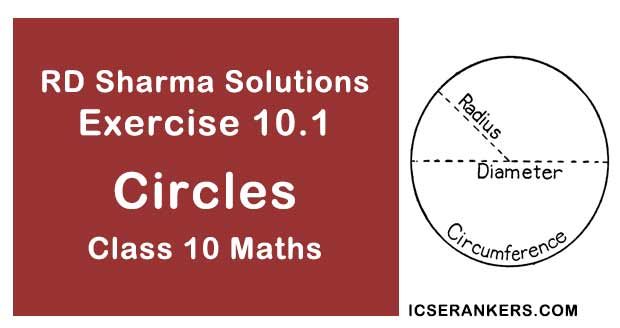Chapter Name RD Sharma Chapter 10 Circles Book Name RD Sharma Mathematics for Class 10 Other Exercises Exercise 10.2 Related Study NCERT Solutions for Class 10 Maths

### Exercise 10.1 Solutions

1. Fill in the blanks
(i) The common point of tangent and the circle is called point of contact.
(ii) A circle may have two parallel tangents.
(iii) A tangent to a circle intersects it in one point.
(iv) A line intersecting a circle in two points A called a secant.
(v)  The angle between tangent at a point P on circle and radius through the point is 90° .

Solution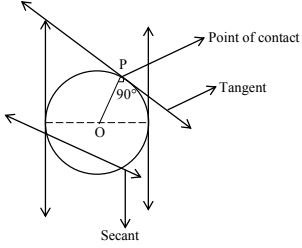2. How many tangents can a circle have ?

Solution

Tangent: A line intersecting circle in one point is called a tangent.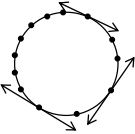As there are infinite number of points on the circle a circle has many (infinite) tangents.

3. O is the center of a circle of radius 8 cm. The tangent at a point A on the circle cuts a line through O at B such that AB = 15 cm. Find OB

Solution

Consider a circle with center O and radius OA = 8 cm = r, AB = 15 cm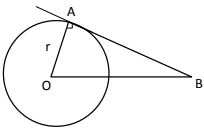(AB) tangent is drawn at A(point of contact)
At point of contact, we know that radius and tangent are perpendicular.
In ΔOAB, ∠OAB = 90°, By Pythagoras theorem4. If the tangent at point P to the circle with center O cuts a line through O at Q such that PQ = 24 cm and OQ = 25 cm. Find the radius of circle.

Solution

Given,
PQ = 24 cm
OQ = 25 cm